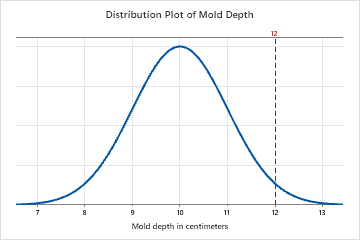# What is a Z-value?

The Z-value is a test statistic for Z-tests that measures the difference between an observed statistic and its hypothesized population parameter in units of the standard deviation. For example, a selection of factory molds has a mean depth of 10cm and a standard deviation of 1 cm. A mold with a depth of 12 cm has a Z-value of 2, because its depth is two standard deviations greater than the mean. The vertical line represents this observation, and its location relative to the entire population:Converting an observation to a Z-value is called standardization. To standardize an observation in a population, subtract the population mean from the observation of interest and divide the result by the population standard deviation. The result of these calculations is the Z-value associated with the observation of interest.

You can use the Z-value to determine whether to reject the null hypothesis. To determine whether to reject the null hypothesis compare the Z-value to your critical value, which can be found in a standard normal table in most statistics books. The critical value is Z1-α/2 for a two sided test and Z1-α for a one sided test. If the absolute value of the Z-value is greater than the critical value, you reject the null hypothesis. If it is not, you fail to reject the null hypothesis.

For example, you want to know whether a second group of molds also has a mean depth of 10cm. You measure the depth of each mold in the second group, and calculate the group's mean depth. A 1-sample Z-test calculates a Z-value of −1.03. You choose an α of 0.05, which results in a critical value of 1.96. Because the absolute value of the Z-value is less than 1.96, you fail to reject the null hypothesis and cannot conclude that the mold's mean depth is different from 10cm.

By using this site you agree to the use of cookies for analytics and personalized content.  Read our policy